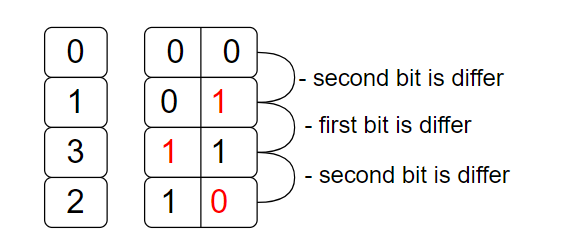New update is available. Click here to update.

# Gray Code

Last Updated: 23 Dec, 2020
Difficulty: Moderate

## PROBLEM STATEMENT

#### Conditions for a gray code sequence :

``````1. Gray code sequence contains numbers from 0 to 2^'grayNumber'-1 in bit/binary form.
2. Two consecutive gray code sequence numbers only differ by 1 bit.
``````

#### Example :

``````Given 'grayNumber' : 2.
````````````As depicted from above image, the gray code sequence is 0,1,3,2.
``````

#### Note :

``````1. The output sequence must contain the decimal representation of numbers instead of the binary form.
2. There can be multiple answers print anyone.
``````
##### Input format :
``````The first line of input contains an integer ‘T’ denoting the number of test cases.

The first line of every test case contains an integer ‘grayNumber’.
``````
##### Output Format :
``````For each test case, return the ‘list/vector’ of the Gray code sequence.

The output is ‘Valid’ if returned gray code sequence is correct. Else ‘Invalid’.
``````
##### Note :
``````You do not need to print anything, it has already been taken care of. Just implement the given function.
``````
##### Constraints :
``````1 <= T <= 2
0 <= grayNumber <= 15

Time Limit : 1 sec
``````## Approach 1

Suppose are finding an answer for ‘N’ size but we have already the solution for ‘N-1’. then use the previous answer for a new answer. (where ‘N’ is ‘grayNumber’)

1. Let’s consider ‘N' : 3 and we have solution of ‘N-1' : 2 : { ‘00’, ‘01’, ‘10’, ‘11’} and called it ‘PRE’.
2. Create a new list/vector of ‘PRE’ in reverse order { ‘11’, ‘10’, ‘01’, ‘00’ } called it ‘NEW’.
3. Add ‘0’ as a prefix, in all the elements of ‘PRE’ : { ‘000’, ‘001’, ‘010’, ‘011’ } /
4. Add ‘1’ as a prefix, in all the elements of ‘NEW’ : { ‘111’, ‘110’, ‘101’, ‘100’} .
5. Concatenate the ‘PRE’ + ‘NEW’ and it will be answered for ‘N' : 3.
6. Return a number conversion of new concatenated ‘list/vector’.
SIMILAR PROBLEMS

JUMP GAME

Posted: 8 Sep, 2022
Difficulty: Moderate

NINJA AND HAPPINESS

Posted: 9 Sep, 2022
Difficulty: Hard

DECODE STRING

Posted: 11 Sep, 2022
Difficulty: Moderate

Randomly Sorted

Posted: 13 Nov, 2022
Difficulty: Moderate

8-Queen Problem

Posted: 19 Dec, 2022
Difficulty: Easy